Test: Network Synthesis - 1

# Test: Network Synthesis - 1

Test Description

## 10 Questions MCQ Test | Test: Network Synthesis - 1

Test: Network Synthesis - 1 for Electrical Engineering (EE) 2023 is part of Electrical Engineering (EE) preparation. The Test: Network Synthesis - 1 questions and answers have been prepared according to the Electrical Engineering (EE) exam syllabus.The Test: Network Synthesis - 1 MCQs are made for Electrical Engineering (EE) 2023 Exam. Find important definitions, questions, notes, meanings, examples, exercises, MCQs and online tests for Test: Network Synthesis - 1 below.
Solutions of Test: Network Synthesis - 1 questions in English are available as part of our course for Electrical Engineering (EE) & Test: Network Synthesis - 1 solutions in Hindi for Electrical Engineering (EE) course. Download more important topics, notes, lectures and mock test series for Electrical Engineering (EE) Exam by signing up for free. Attempt Test: Network Synthesis - 1 | 10 questions in 30 minutes | Mock test for Electrical Engineering (EE) preparation | Free important questions MCQ to study for Electrical Engineering (EE) Exam | Download free PDF with solutions
 1 Crore+ students have signed up on EduRev. Have you?
Test: Network Synthesis - 1 - Question 1

### Which of the following is a positive real function?

Detailed Solution for Test: Network Synthesis - 1 - Question 1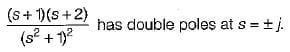Hence, notaPRF.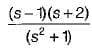has a zero (s = 1) in RH s-plane.

Hence, not a PRF.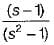has a pole and a zero in RH s-piane.
Hence, not a PRF.
Option (b) is a PRF as all poles and zeros lie in LH s-plane.

Test: Network Synthesis - 1 - Question 2

### A network function can be completely specified by:

Test: Network Synthesis - 1 - Question 3

### Which of the following property does not relates to LC impedance or admittance functions?

Test: Network Synthesis - 1 - Question 4

If the impulse response is realisable by delaying it appropriately and is bounded for bounded excitation, then the system is said to be

Detailed Solution for Test: Network Synthesis - 1 - Question 4

Since system is bounded for bounded excitation therefore, it will be stable.
Since the system is delayed therefore, output may exist before the input is applied.
Hence, the system will be non-causal.

Test: Network Synthesis - 1 - Question 5

A network-A has zeros only in the left-half of the s-plane while the network-B has zeros only in the right-half of the s-plane. The network-A and Network-B will be respectively called

Test: Network Synthesis - 1 - Question 6

An L-C admittance or impedance function

Test: Network Synthesis - 1 - Question 7

If F1(s) and F2(s) are positive real functions, then which of the following are positive real function?

Test: Network Synthesis - 1 - Question 8

For the pole-zero plot shown below, the network function is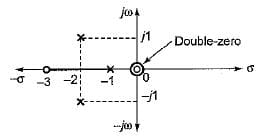Detailed Solution for Test: Network Synthesis - 1 - Question 8

Here, zeros are at: s = 0, 0,-3

Poles are at s = - 1,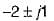or, poles are at (s + 2)2 + 1 - 0 and at s = -1 or, s2 + 4s + 5 = 0 and at s = -1

Test: Network Synthesis - 1 - Question 9

A stable system must have

Test: Network Synthesis - 1 - Question 10

Which of the following is not a positive real function?

Detailed Solution for Test: Network Synthesis - 1 - Question 10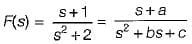Here, a = 1 , b = 0, c = 2

Thus, a, b, c ≥ 0 but b < a

For PRF, b ≥ a therefore, option (d) is not a PRF.

 Use Code STAYHOME200 and get INR 200 additional OFF Use Coupon Code
Information about Test: Network Synthesis - 1 Page
In this test you can find the Exam questions for Test: Network Synthesis - 1 solved & explained in the simplest way possible. Besides giving Questions and answers for Test: Network Synthesis - 1, EduRev gives you an ample number of Online tests for practice